# Generate Reward Function from a Model Predictive Controller for a Servomotor

This example shows how to automatically generate a reward function from cost and constraint specifications defined in a model predictive controller object. You then use the generated reward function to train a reinforcement learning agent.

### Introduction

You can use the `generateRewardFunction` to generate a reward function for reinforcement learning, starting from cost and constraints specified in a model predictive controller. The resulting reward signal is a sum of costs (as defined by an objective function) and constraint violation penalties depending on the current state of the environment.

This example is based on theDC Servomotor with Constraint on Unmeasured Output (Model Predictive Control Toolbox) example, in which you design a model predictive controller for a DC servomechanism under voltage and shaft torque constraints. Here, you will convert the cost and constraints specifications defined in the `mpc` object into a reward function and use it to train an agent to control the servomotor.

Open the Simulink model for this example which is based on the above MPC example but has been modified for reinforcement learning.

`open_system('rl_motor')`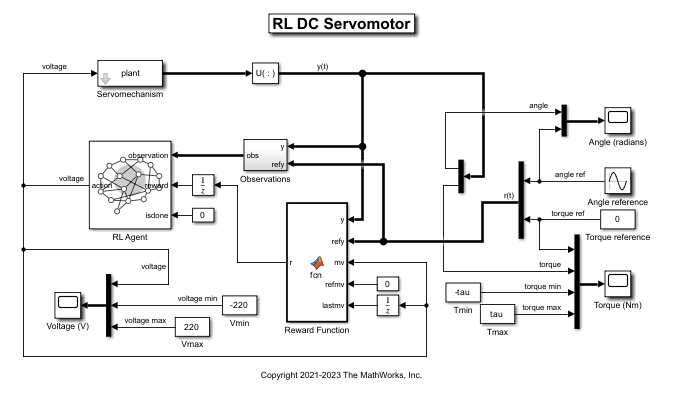### Create Model Predictive Controller

Create the open-loop dynamic model of the motor, defined in `plant` and the maximum admissible torque `tau` using an helper function.

`[plant,tau] = mpcmotormodel;`

Specify input and output signal types for the MPC controller. The shaft angular position, is measured as first output. The second output, torque, is unmeasurable.

`plant = setmpcsignals(plant,'MV',1,'MO',1,'UO',2);`

Specify constraints on the manipulated variable, and define a scale factor.

`MV = struct('Min',-220,'Max',220,'ScaleFactor',440);`

Impose torque constraints during the first three prediction steps, and specify scale factor for both shaft position and torque.

```OV = struct('Min',{-Inf, [-tau;-tau;-tau;-Inf]},... 'Max',{Inf, [tau;tau;tau;Inf]},... 'ScaleFactor',{2*pi, 2*tau});```

Specify weights for the quadratic cost function to achieve angular position tracking. Set to zero the weight for the torque, thereby allowing it to float within its constraint.

`Weights = struct('MV',0,'MVRate',0.1,'OV',[0.1 0]);`

Create an MPC controller for the `plant` model with a sample time of `0.1` s, a prediction horizon `10` steps, and a control horizon of `2` steps, using the previously defined structures for the weights, manipulated variables, and output variables.

`mpcobj = mpc(plant,0.1,10,2,Weights,MV,OV);`

Display the controller specifications.

`mpcobj`
``` MPC object (created on 26-Feb-2022 15:07:51): --------------------------------------------- Sampling time: 0.1 (seconds) Prediction Horizon: 10 Control Horizon: 2 Plant Model: -------------- 1 manipulated variable(s) -->| 4 states | | |--> 1 measured output(s) 0 measured disturbance(s) -->| 1 inputs | | |--> 1 unmeasured output(s) 0 unmeasured disturbance(s) -->| 2 outputs | -------------- Indices: (input vector) Manipulated variables: [1 ] (output vector) Measured outputs: [1 ] Unmeasured outputs: [2 ] Disturbance and Noise Models: Output disturbance model: default (type "getoutdist(mpcobj)" for details) Measurement noise model: default (unity gain after scaling) Weights: ManipulatedVariables: 0 ManipulatedVariablesRate: 0.1000 OutputVariables: [0.1000 0] ECR: 10000 State Estimation: Default Kalman Filter (type "getEstimator(mpcobj)" for details) Constraints: -220 <= MV1 (V) <= 220, MV1/rate (V) is unconstrained, MO1 (rad) is unconstrained -78.54 <= UO1 (Nm)(t+1) <= 78.54 -78.54 <= UO1 (Nm)(t+2) <= 78.54 -78.54 <= UO1 (Nm)(t+3) <= 78.54 UO1 (Nm)(t+4) is unconstrained ```

The controller operates on a plant with 4 states, 1 input (voltage) and 2 output signals (angle and torque) and has the following specifications:

• The cost function weights for the manipulated variable, manipulated variable rate and output variables are 0, 0.1 and [0.1 0] respectively.

• The manipulated variable is constrained between -220V and 220V.

• The manipulated variable rate is unconstrained.

• The first output variable (angle) is unconstrained but the second (torque) is constrained between -78.54 Nm and 78.54 Nm in the first three prediction time steps and unconstrained in the fourth step.

Note that for reinforcement learning only the constraints specification from the first prediction time step will be used since the reward is computed for a single time step.

### Generate the Reward Function

Generate the reward function code from specifications in the `mpc` object using `generateRewardFunction`. The code is displayed in the MATLAB Editor.

```generateRewardFunction(mpcobj) ```

The generated reward function is a starting point for reward design. You can modify the function with different penalty function choices and tune the weights. For this example, make the following change to the generated code:

• Scale the original cost weights `Qy`, `Qmv` and `Qmvrate` by a factor of 100.

• The default exterior penalty function method is `step`. Change the method to `quadratic`.

After you make changes, the cost and penalty specifications should be as follows:

```Qy = [10 0]; Qmv = 0; Qmvrate = 10; Py = Wy * exteriorPenalty(y,ymin,ymax,'quadratic'); Pmv = Wmv * exteriorPenalty(mv,mvmin,mvmax,'quadratic'); Pmvrate = Wmvrate * exteriorPenalty(mv-lastmv,mvratemin,mvratemax,'quadratic'); ```

For this example, the modified code has been saved in the MATLAB function file `rewardFunctionMpc.m`. Display the generated reward function.

`type rewardFunctionMpc.m`
```function reward = rewardFunctionMpc(y,refy,mv,refmv,lastmv) % REWARDFUNCTIONMPC generates rewards from MPC specifications. % % Description of input arguments: % % y : Output variable from plant at step k+1 % refy : Reference output variable at step k+1 % mv : Manipulated variable at step k % refmv : Reference manipulated variable at step k % lastmv : Manipulated variable at step k-1 % % Limitations (MPC and NLMPC): % - Reward computed based on first step in prediction horizon. % Therefore, signal previewing and control horizon settings are ignored. % - Online cost and constraint update is not supported. % - Custom cost and constraint specifications are not considered. % - Time varying cost weights and constraints are not supported. % - Mixed constraint specifications are not considered (for the MPC case). % Reinforcement Learning Toolbox % 02-Jun-2021 16:05:41 %#codegen %% Specifications from MPC object % Standard linear bounds as specified in 'States', 'OutputVariables', and % 'ManipulatedVariables' properties ymin = [-Inf -78.5398163397448]; ymax = [Inf 78.5398163397448]; mvmin = -220; mvmax = 220; mvratemin = -Inf; mvratemax = Inf; % Scale factors as specified in 'States', 'OutputVariables', and % 'ManipulatedVariables' properties Sy = [6.28318530717959 157.07963267949]; Smv = 440; % Standard cost weights as specified in 'Weights' property Qy = [0.1 0]; Qmv = 0; Qmvrate = 0.1; %% Compute cost dy = (refy(:)-y(:)) ./ Sy'; dmv = (refmv(:)-mv(:)) ./ Smv'; dmvrate = (mv(:)-lastmv(:)) ./ Smv'; Jy = dy' * diag(Qy.^2) * dy; Jmv = dmv' * diag(Qmv.^2) * dmv; Jmvrate = dmvrate' * diag(Qmvrate.^2) * dmvrate; Cost = Jy + Jmv + Jmvrate; %% Penalty function weight (specify nonnegative) Wy = [1 1]; Wmv = 10; Wmvrate = 10; %% Compute penalty % Penalty is computed for violation of linear bound constraints. % % To compute exterior bound penalty, use the exteriorPenalty function and % specify the penalty method as 'step' or 'quadratic'. % % Alternatively, use the hyperbolicPenalty or barrierPenalty function for % computing hyperbolic and barrier penalties. % % For more information, see help for these functions. % % Set Pmv value to 0 if the RL agent action specification has % appropriate 'LowerLimit' and 'UpperLimit' values. Py = Wy * exteriorPenalty(y,ymin,ymax,'step'); Pmv = Wmv * exteriorPenalty(mv,mvmin,mvmax,'step'); Pmvrate = Wmvrate * exteriorPenalty(mv-lastmv,mvratemin,mvratemax,'step'); Penalty = Py + Pmv + Pmvrate; %% Compute reward reward = -(Cost + Penalty); end ```

To integrate this reward function, open the MATLAB Function block in the Simulink model.

`open_system('rl_motor/Reward Function')`

Append the function with the following line of code and save the model.

`r = rewardFunctionMpc(y,refy,mv,refmv,lastmv);`

The MATLAB Function block will now execute `rewardFunctionMpc.m` during simulation.

For this example, the MATLAB Function block has already been modified and saved.

### Create a Reinforcement Learning Environment

The environment dynamics are modeled in the Servomechanism subsystem. For this environment,

• The observations are the reference and actual output variables (angle and torque) from the last 8 time steps.

• The action is the voltage $\mathit{V}$ applied to the servomotor.

• The sample time is ${\mathit{T}}_{\mathit{s}}=0.1\mathit{s}$.

• The total simulation time is ${\mathit{T}}_{\mathit{f}}=20\mathit{s}$.

Specify the total simulation time and sample time.

```Tf = 20; Ts = 0.1;```

Create observation and action specifications for the environment.

```numObs = 32; numAct = 1; oinfo = rlNumericSpec([numObs 1]); ainfo = rlNumericSpec([numAct 1],'LowerLimit',-220,'UpperLimit',220);```

Create the reinforcement learning environment using the `rlSimulinkEnv` function.

`env = rlSimulinkEnv('rl_motor','rl_motor/RL Agent',oinfo,ainfo);`

#### Create a Reinforcement Learning Agent

Fix the random seed for reproducibility.

`rng(0)`

The agent in this example is a Twin Delayed Deep Deterministic Policy Gradient (TD3) agent.

Create two critic representations.

```% Critic cnet = [ featureInputLayer(numObs,'Normalization','none','Name', 'State') fullyConnectedLayer(128, 'Name', 'fc1') concatenationLayer(1,2,'Name','concat') reluLayer('Name','relu1') fullyConnectedLayer(64, 'Name', 'fc3') reluLayer('Name','relu2') fullyConnectedLayer(1, 'Name', 'CriticOutput')]; actionPath = [ featureInputLayer(numAct,'Normalization','none', 'Name', 'Action') fullyConnectedLayer(8, 'Name', 'fc2')]; criticNetwork = layerGraph(cnet); criticNetwork = addLayers(criticNetwork, actionPath); criticNetwork = connectLayers(criticNetwork,'fc2','concat/in2'); criticOptions = rlRepresentationOptions('LearnRate',1e-3,'GradientThreshold',1); critic1 = rlQValueRepresentation(criticNetwork,oinfo,ainfo,... 'Observation',{'State'},'Action',{'Action'},criticOptions); critic2 = rlQValueRepresentation(criticNetwork,oinfo,ainfo,... 'Observation',{'State'},'Action',{'Action'},criticOptions);```

Create an actor representation.

```actorNetwork = [ featureInputLayer(numObs,'Normalization','none','Name','State') fullyConnectedLayer(128, 'Name','actorFC1') reluLayer('Name','relu1') fullyConnectedLayer(64, 'Name','actorFC2') reluLayer('Name','relu2') fullyConnectedLayer(numAct,'Name','Action')]; actorOptions = rlRepresentationOptions('LearnRate',1e-3,'GradientThreshold',1); actor = rlDeterministicActorRepresentation(actorNetwork,oinfo,ainfo,... 'Observation',{'State'},'Action',{'Action'},actorOptions);```

Specify the agent options using `rlTD3AgentOptions`. The agent trains from an experience buffer of maximum capacity `1e6` by randomly selecting mini-batches of size `256`. The discount factor of `0.995` favors long-term rewards.

```agentOpts = rlTD3AgentOptions("SampleTime",Ts, ... "DiscountFactor", 0.995, ... "ExperienceBufferLength",1e6, ... "MiniBatchSize",256);```

The exploration model in this TD3 agent is Gaussian. The noise model adds a uniform random value to the action during training. Set the standard deviation of the noise to `100`. The standard deviation decays at the rate of `1e-5` every agent step until the minimum value of `0.005`.

```agentOpts.ExplorationModel.StandardDeviationMin = 0.005; agentOpts.ExplorationModel.StandardDeviation = 100; agentOpts.ExplorationModel.StandardDeviationDecayRate = 1e-5;```

Create the TD3 agent using the actor and critic representations. For more information on TD3 agents, see `rlTD3Agent`.

`agent = rlTD3Agent(actor,[critic1,critic2],agentOpts);`

#### Train the Agent

To train the agent, first specify the training options using `rlTrainingOptions`. For this example, use the following options:

• Run each training for at most 2000 episodes, with each episode lasting at most `ceil(Tf/Ts)` time steps.

• Stop the training when the agent receives an average cumulative reward greater than `-2` over `20` consecutive episodes. At this point, the agent can track the reference signal.

```trainOpts = rlTrainingOptions(... 'MaxEpisodes',2000, ... 'MaxStepsPerEpisode',ceil(Tf/Ts), ... 'StopTrainingCriteria','AverageReward',... 'StopTrainingValue',-2,... 'ScoreAveragingWindowLength',20);```

Train the agent using the `train` function. Training this agent is a computationally intensive process that may take several minutes to complete. To save time while running this example, load a pretrained agent by setting `doTraining` to `false`. To train the agent yourself, set `doTraining` to `true`.

```doTraining = false; if doTraining trainingStats = train(agent,env,trainOpts); else load('rlDCServomotorTD3Agent.mat') end```

A snapshot of the training progress is shown in the following figure. You can expect different results due to inherent randomness in the training process.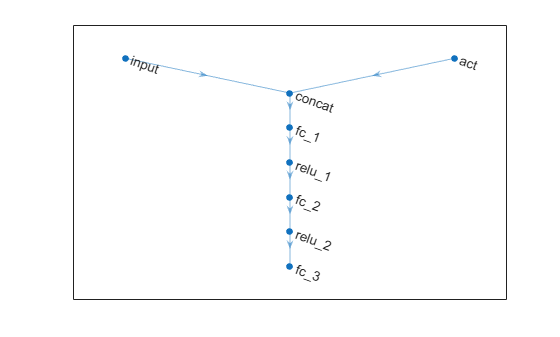#### Validate Controller Response

To validate the performance of the trained agent, simulate the model and view the response in the Scope blocks. The reinforcement learning agent is able to track the reference angle while satisfying the constraints on torque and voltage.

`sim('rl_motor');`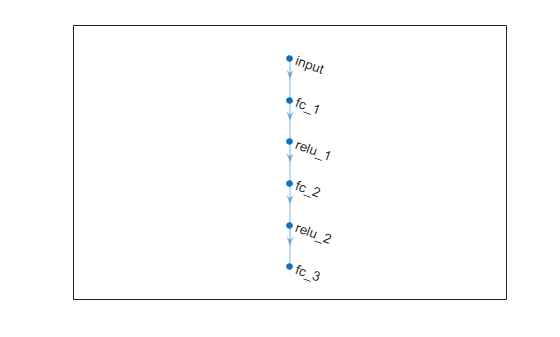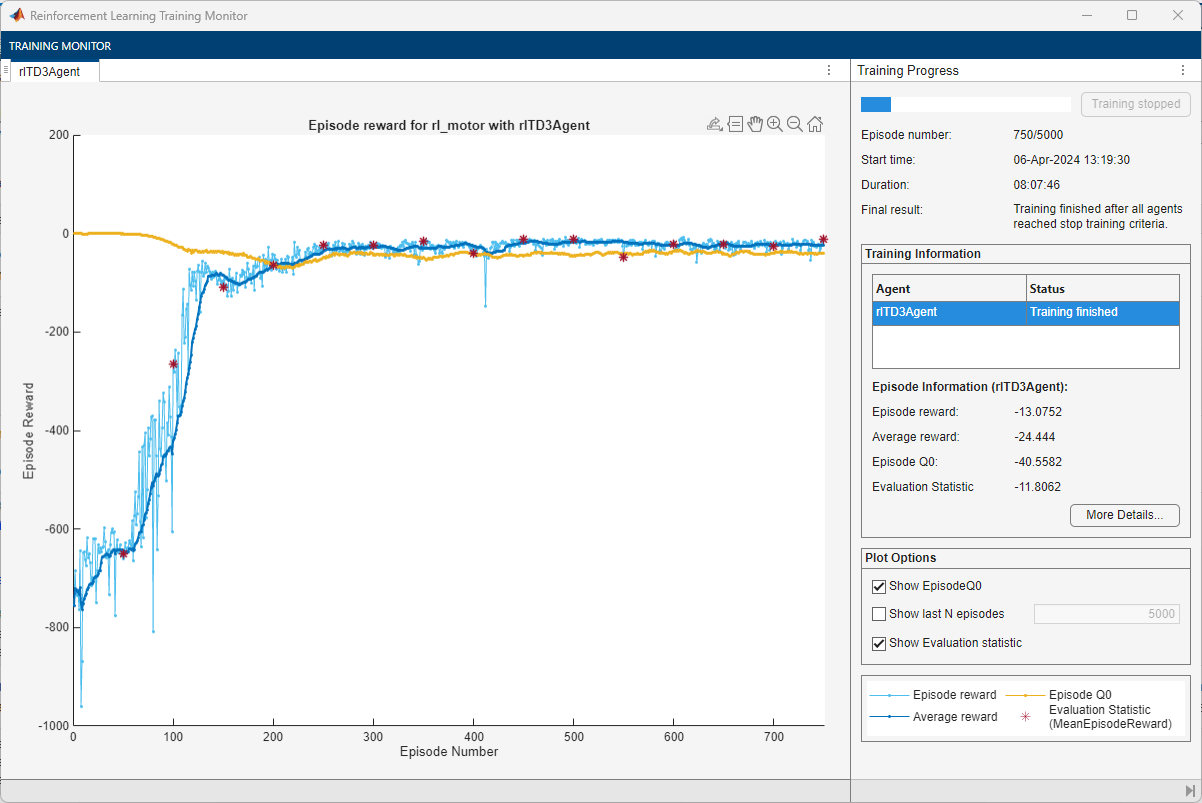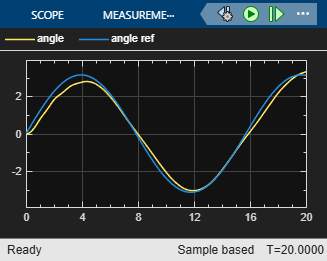Close the model.

`close_system('rl_motor')`